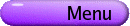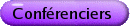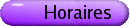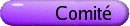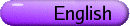Conférenciers pléniers[PDF] ROBERT GURALNICK, University of Southern California Probabilistic Methods in Finite Group Theory [PDF] We shall discuss the use of probabilistic methods in generation of finite groups. We shall give a survey of some of the main results in the area and also discuss some more recent results. We shall also discuss some related results on derangements in finite primitive permutation groups. UFFE HAAGERUP, South Denmark University Free probability and the invariant subspace problem for von Neumann algebras [PDF] Does every operator T on a Hilbert space H have a non-trivial closed invariant subspace? This is the famous and still open "invariant subspace problem" for operators on a Hilbert space. A natural generalization of the problem is: Let M be a von Neumann algebra on a Hilbert space H. Does every operator T in M have a non-trivial closed invariant subspace K affiliated with M? (K is affiliated with M, iff the orthogonal projection on K belongs to M.) In the special case, when M is a II1-factor (i.e., a infinite dimensional von Neumann factor with a bounded trace), it turns out, that "almost all" operators in M have non-trivial closed invariant subspaces affiliated with M. More precisely, it holds for all operators in M for which L. G. Brown's spectral distribution measure for T is not concentated in a single point of the complex plane. The result is obtained in collaboration with Hanne Schultz, and it relies in a crucial way on Voiculescu's free probability theory. BRYNA KRA, Northwestern University Additive Combinatorics and Ergodic Theory [PDF] A beautiful result in combinatorial number theory is Szemeredi's Theorem: a set of integers with positive upper density contains arbitrarily long arithmetic progressions. In the 1970s, Furstenberg established the deep connections between combinatorics and ergodic theory, using ergodic theory to prove Szemeredi's Theorem. This development lead to the field of Ergodic Ramsey Theory and many new combinatorial and number theoretic statements were proven using ergodic theory. In the last year, this interaction took a new twist, with ergodic methods playing an important role in Green and Tao's proof that the prime numbers contain arbitrarily long arithmetic progressions. I will give an overview of this interplay, with a focus on recent developments in ergodic theory. ANDREW MAJDA, New York University, Courant Institute, 251 Mercer St., NY, NY 10012 New waves, PDEs, and coarse-grained stochastic lattice models for the tropics [PDF] One of the most important and prototype multi-scale systems involves a comprehensive model for the coupled atmosphere ocean system for both climate change and longer term weather prediction. One of the striking recent observational discoveries is the profound impact of variations in the tropics on all of these issues. The talk has four parts: (1) an introduction to these issues; (2) novel behavior of waves in the simplest tropical climate models and their mathematical analysis compared/contrasted with relaxation limits and combustion waves; (3) systematic mathematical strategies for coarse graining stochastic lattice models for both material science and climate physics; (4) application of (3) to show the dramatic effect in simple tropical climate models of stochastic effects on both the climatology and fluctuations. ODED SCHRAMM, Microsoft Understanding 2D critical percolation: from Harris to Smirnov and beyond [PDF] There are numerous predictions from statistical physics regarding random systems in the plane which were until recently beyond the reach of mathematical understanding. Some of the better-known examples include percolation and the Ising model. We will focus on percolation and describe our growing understanding of it through a sequence of insights (which are simple in hindsight) from 1960 through today.